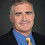## Thursday, April 04, 2013

### Gary Miller to Receive the Knuth Prize

The 2013 Knuth Prize will be awarded to Gary Miller at STOC (ACM Press Release). The Knuth prize is now given yearly for outstanding contributions to the foundations of computer science, jointly sponsored by ACM SIGACT and the IEEE TC on Mathematical Foundations of Computer Science.

In 1975, Gary Miller gave a polynomial-time algorithm for primality assuming that the extended Riemann Hypothesis is true. For a given n, if there is an x and j such that x2j mod n is not 1 or -1 and x2j+1 mod n ≡ 1 then n can't be prime. Gary showed that ERH implied that if n is composite there must be such an x ≤ O(log2 n). Given ERH one could just search all possible x and j in time polynomial in the number of bits to describe n.

Rabin noted that one could choose x at random to get a probabilistic algorithm that was correct with high confidence without any ERH assumption, now called the Miller-Rabin test. In 2002, Agrawal, Kayal and Saxena showed a polynomial-time algorithm for primality without assumption, but the Miller-Rabin test is still much faster in practice.

This is just part of Miller's contribution. From the press release:

Miller also made significant contributions to the theory of isomorphism testing—the problem of telling whether two structures are the same except for the labeling of their components.  He showed the equivalence of many different isomorphism problems to the still-open problem of graph isomorphism, and identified many special cases that could be solved efficiently. These included the problem of testing isomorphism for a special case known as bounded-genus graphs, a result he obtained with John Reif in 1980.  In 1985, in another collaboration with Reif, Miller invented the concept of "parallel tree contraction." This is one of the most fundamental primitives in parallel algorithm design with wide applications to graph theoretical and algebraic problems.

In 1984, Miller moved into the area of scientific computing. He set up the theoretical foundations for mesh generation, and was the first to design meshing algorithms with near-optimal runtime guarantees.  His subsequent research led to his breakthrough 2010 results with Ioannis Koutis and Richard Peng that currently provide the fastest algorithms—in theory and practice—for solving "symmetric diagonally dominant" linear systems. These systems have important applications in image processing, network algorithms, engineering, and physical simulations.

Come to STOC and see Gary Miller's Knuth prize lecture. The program has just been posted and if you need financial help to attend, the student travel grant deadline is April 16.

1.1.2.